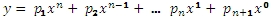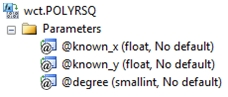# SQL Server polynomial goodness-of-fit

POLYRSQ

Updated: 30 April 2011

Use POLYRSQ for calculating the goodness of fit for an approximating polynomial calculated from a series of x- and y-values for a specified number of degrees. The goodness of fit is calculated using the coefficients of a polynomial p(x) of degree n that fits the x- and y-values supplied to the function. The y-value is calculated using n+1 polynomial coefficients in descending powers:We can think of the POLYRSQ value as the fraction of variance explained by the approximating polynomial. Thus, it becomes the ratio of the variance of the y-values input to the function and the y-values calculated by the approximating polynomial.
SyntaxArguments
@known_x
the x-values to be used in the calculation. @known_x must be of the type float or of a type that implicitly converts to float.
@known_y
the y-values to be used in the calculation. @known_y must be of the type float or of a type that implicitly converts to float.
@degree
an integer specifying the degree of the polynomial. @degree must be of the type samllint or of a type that implicitly converts to samllint.
Return Types
float
Remarks
·         The x- and y-values are passed to the function as pairs
·         If x is NULL or y is NULL, the pair is not used in the calculation.
·         @degree must be less than the number of x-y rows in the GROUP.
·         POLYRSQ returns a value between 0 and 1.
·         @degree must remain invariant for the GROUP.
·         Available in XLeratorDB / math 2008 only
Examples
In this example, we will use the westclintech SERIESFLOAT function to generate a series of x-values equally spaced in the interval [0, 2.5] and then evaluate the error function, ERF, at those points. We will specify an approximating polynomial of 6 degrees. We will use the POLYRSQ function to determine the goodness of fit of the approximating polynomial in the specified interval
SET NOCOUNT ON

SELECT wct.POLYRSQ(x,y,6) as [R-squared]
FROM (
SELECT SeriesValue as x
,westclintech.wct.ERF(SeriesValue) as y
FROM wct.SeriesFloat(0,2.5,0.1,NULL,NULL)
) n
This produces the following result.
R-squared
----------------------
0.999999133147865

This leads us to believe that in the interval [0, 2.5] that the 6-degree approximating polynomial is in fact a very good fit to the function.
What if we had chosen a polynomial of lesser degree? In this example, we will use the same data, but we will set the degree to 3 rather than 6.
SET NOCOUNT ON

SELECT wct.POLYRSQ(x,y,3) as [R-squared]
FROM (
SELECT SeriesValue as x
,westclintech.wct.ERF(SeriesValue) as y
FROM wct.SeriesFloat(0,2.5,0.1,NULL,NULL)
) n
This produces the following result.
R-squared
----------------------
0.999459358730253

It is important to note that the r-squared statistic does not tell us anything about the goodness of fit beyond the bounds of the interval passed into the function. In other words, for this example, if we wanted to calculate new y-values for x where x is in the interval [0, 2.5], the r-squared statistic is telling us that the calculated value using the approximating polynomial would be a pretty good fit to the function. However, if we used the approximating polynomial for x less than zero or x greater than 2.5, then we really don’t know how good the fit will be.

In this example, we will look at a yield curve which contains yield values for years 1, 2, 3, 5, 7, 10, 20, and 30. We will calculate the R-squared value for approximating polynomials for degrees 1 through 6.
SET NOCOUNT ON

SELECT a.degree as [degree]
,wct.POLYRSQ(x,y,a.degree) as [R-squared]
FROM (values
(1, .0028),
(2, .0056),
(3, .0085),
(5, .0164),
(7, .0235),
(10, .0299),
(20, .0382),
(30, .0406)
) n(x, y)
CROSS APPLY
(VALUES (1),(2),(3),(4),(5),(6)) a(degree)
GROUP BY a.degree
This produces the following result.
degree              R-squared
----------- ----------------------
1      0.827136195741199
2      0.984674406456717
3      0.996593232419546
4      0.998611850657441
5      0.999909161372577
6      0.999947928815932

We can see that in this case as the degree of the approximating polynomial increases, the R-squared value increases, meaning that the goodness of fit improves as the degree increases. Thus, if we were to calculate a new y-value in the interval between 1 and 30 (years), a third or fourth degree polynomial is probably adequate.

The POLYRSQ can be thought of as the results of RSQ calculation from the XLeratorDB/statistics module, using the known y-values and the calculated y-values. In this example, we will use the POLYVAL function to generate new y-values using a third-degree polynomial and then apply the RSQ function to the
results.
SET NOCOUNT ON

SELECT *
INTO #xy
FROM (values
(1, .0028),
(2, .0056),
(3, .0085),
(5, .0164),
(7, .0235),
(10, .0299),
(20, .0382),
(30, .0406)
) n(x, y)

SELECT wctStatistics.wct.RSQ(y,f) as [R-squared]
FROM (
SELECT a.x
,a.y
,wct.POLYVAL(b.x, b.y, 3, a.x) as f
FROM #xy a, #xy b
GROUP BY a.x
,a.y
) n

DROP TABLE #xy
This produces the following result.
R-squared
----------------------
0.996593232419697

Which is the same answer produced by POLYRSQ out to 14 significant digits.

### SupportCopyright 2008-2021 Westclintech LLC         Privacy Policy        Terms of Service